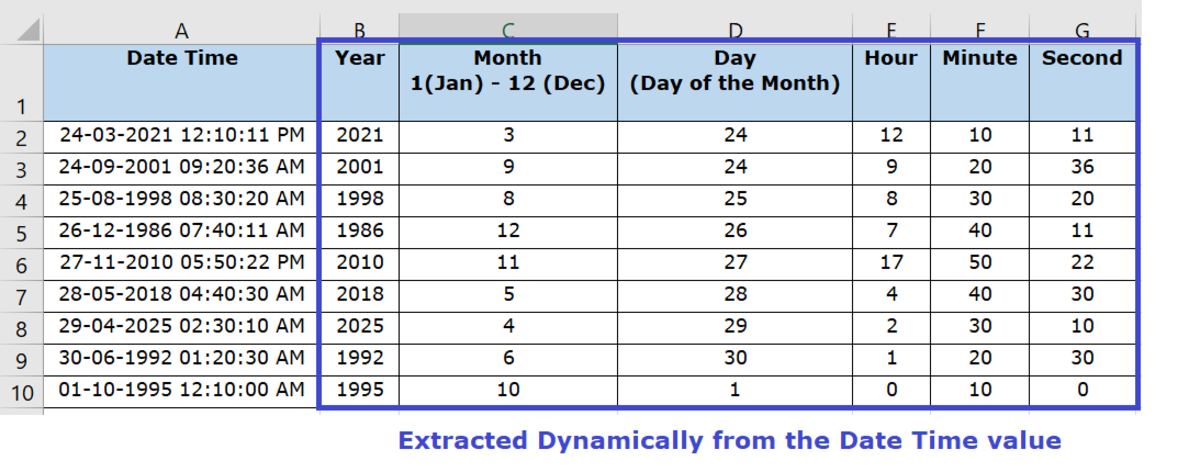# How to Dynamically Extract the Year, Month, Day, Hour, Minute, and Second From a Date-Time Value in Excel

Neha is a software professional with 13+ years of experience in the IT Industry. She enjoys writing technical tutorials.How to Dynamically Extract the Year, Month, Day, Hour, Minute and Second From a Date-Time Value in Excel

## How Do I Extract the Year, Month, Day, etc. From a Date-Time Value? (3 Easy Steps)

Do you want to learn how to dynamically extract the year, month, day, hour, minute, and second from a date-time value in Excel? This is also called "date stripping", and I will teach you how!

Here's my step-by-step tutorial with easy instructions to follow.

### 1. Identify the Date-Time Field Where You Would Like to Extract These Values

Let us assume we have a date-time value in a Microsoft Excel Worksheet and we would like to dynamically extract the year, month, day, hour, minute, and second from that date-time value.

Here's the sample data we are going to use to demonstrate how to do this.

In our table here, we have a list of date-time values in the (DD-MM-YYYY HH:MM:SS AM/PM) format. We are going to use Microsoft Excel formulas to dynamically extract the individual values for the year, month, day, hour, minute, and second.

So in this case, the column to be used to determine these values would be Column A, that is holding the date-time values.

### 2. Use the Formula in the Cell Where You Want the Extracted Value to Appear

Here's how we're going to do this part, step by step.

### Year

Formula to be entered in cell: B2 (That's where the Year should appear)

Formula to be used: YEAR (serial_number)—Returns the year of a date, an integer in the range of 1900–9999.

### Month

Formula to be entered in the cell: C2 (That's where the Month should appear).

Formula to be used: MONTH (serial_number)—Returns the month, a number from 1 (January) to 12 (December).

### Day

Formula to be entered in the cell: D2 (That's where the Day should appear).

Formula to be used: DAY (serial_number)—Returns the date of the month, a number from 1 to 31.

Scroll to Continue

### Hour

Formula to be entered in cell: D2 (That's where the Hour should appear).

Formula to be used: HOUR (serial_number)—Returns the hour as a number from 0 (12:00 AM) to 23 (11:00 PM).

### Minute

Formula to be entered in the cell: E2 (That's where the Minute should appear).

Formula to be used: MINUTE (serial_number)—Returns the minute, a number from 0 to 59.

### Second

Formula to be entered in the cell: E2 (That's where the Second should appear).

Formula to be used: SECOND (serial_number)—Returns the second, a number from 0 to 59.

Keep in Mind:

For all the above formulae, serial_number is the column that is holding the date-time value. So in this case, A2.

### 3. Copy the Formula to the Remaining Cells

You could either copy and paste the formula in the remaining cells, or you could drag the plus sign at the bottom right corner of the cell so that the formula gets copied to the remaining cells.

### And, Done!

Once the formula is copied to the other cells, it automatically extracts the values from the entire list of the date-time values.

The result is the year, month, day, hour, minute, and second value for each of the date-time values in the selected column.